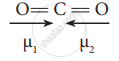Tamil Nadu Board of Secondary EducationHSC Science Class 11th

# Linear form of carbondioxide molecule has two polar bonds. Yet the molecule has Zero dipole moment. Why? - Chemistry

Linear form of carbondioxide molecule has two polar bonds. Yet the molecule has Zero dipole moment. Why?

#### Solution

The linear form of carbon dioxide has zero dipole moment, even though it has two polar bonds. In CO2, the dipole moments of two polar bonds (CO) are equal in magnitude but have opposite direction. Hence, the net dipole moment of the CO2 is,

μ = μ1 + μ2 = μ1 + (-μ1) = 0In this case μ = vec mu_1 + vec mu_2

= vec mu_1 + vec ((-mu_1) = 0

Concept: Bond Parameters
Is there an error in this question or solution?

#### APPEARS IN

Tamil Nadu Board Samacheer Kalvi Class 11th Chemistry Volume 1 and 2 Answers Guide
Chapter 10 Chemical bonding
Evaluation | Q I. 10. | Page 107
Share